# High School Chemistry : Using Scientific Notation

## Example Questions

← Previous 1

### Example Question #1 : Perform Operations With Numbers Expressed In Scientific Notation: Ccss.Math.Content.8.Ee.A.4

Convert the given value to scientific notation.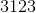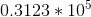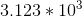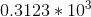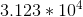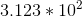Explanation:

Scientific notation is used to simplify exceptionally complex numbers and to quickly present the number of significant figures in a given value. The value is converted to an exponent form using base ten, such that only a single-digit term with any given number of decimal places is used to represent the significant figures of the given value. Non-significant zeroes can be omitted from the leading term, and represented only in the base ten exponent.

The given number has four significant figures (3123), so there will be four digits multiplied by the base ten term.

To generate the single-digit leading term the decimal must be placed after the first 3. Then count the digits to the right of the decimal (123). Our ten will be raised to the power of three.

Our final answer is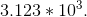### Example Question #1 : Scientific Notation And Significant Figures

Convert the given value to scientific notation.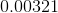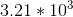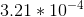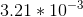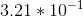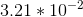Explanation:

Scientific notation is used to simplify exceptionally complex numbers and to quickly present the number of significant figures in a given value. The value is converted to an exponent form using base ten, such that only a single-digit term with any given number of decimal places is used to represent the significant figures of the given value. Non-significant zeroes can be omitted from the leading term, and represented only in the base ten exponent.

The given number has three significant figures (321), so there will be three digits multiplied by the base ten term.

To generate the single-digit leading term the decimal must be placed after the 3 (3.21). Then count the digits to the left of the decimal (moving from right to left, these are 3, 0, 0). Our ten will be raised to the power of negative three because there are three digits to the left of the final decimal placement.

Our final answer is.

### Example Question #1 : Scientific Notation And Significant Figures

Convert the given value to scientific notation.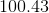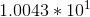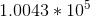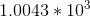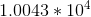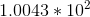Explanation:

Scientific notation is used to simplify exceptionally complex numbers and to quickly present the number of significant figures in a given value. The value is converted to an exponent form using base ten, such that only a single-digit term with any given number of decimal places is used to represent the significant figures of the given value. Non-significant zeroes can be omitted from the leading term, and represented only in the base ten exponent.

The given number has five significant figures (100.43), so there will be five digits multiplied by the base ten term.

To generate the single-digit leading term the decimal must be placed after the 1 (1.0043). Then count the digits to the right of the decimal to determine the change in decimal placement (the decimal moves past the two zeroes). Our ten will be raised to the power of two because there are two digits to the right of the final decimal placement.

Our final answer is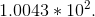### Example Question #1 : Scientific Notation And Significant Figures

Convert the given value to scientific notation.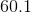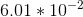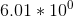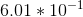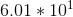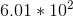Explanation:

Scientific notation is used to simplify exceptionally complex numbers and to quickly present the number of significant figures in a given value. The value is converted to an exponent form using base ten, such that only a single-digit term with any given number of decimal places is used to represent the significant figures of the given value. Non-significant zeroes can be omitted from the leading term, and represented only in the base ten exponent.

The given number has three significant figures (60.1), so there will be three digits multiplied by the base ten term.

To generate the single-digit leading term the decimal must be placed after the 6 (6.01). Then count the digits to the right of the decimal to determine the change in decimal placement (the decimal moves past the zero only). Our ten will be raised to the power of one because there is only one digit to the right of the final decimal placement.

Our final answer is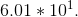### Example Question #31 : High School Chemistry

Convert the given value to standard notation.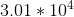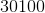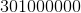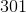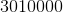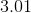Explanation:

Converting a value from scientific notation to standard notation is a simple matter of multiplication.Since we are always multiplying by a base ten term, the value of the exponent determines the increase/decrease in terms of orders of magnitude. In this case, an exponent of 4 indicates a four-fold increase in order of magnitude. Some may find it easiest to determine the answer by simply adjusting the decimal four digits to the right, adding zeroes as necessary.

Whether you choose to multiply or to simply shift the decimal, the final answer will be.

### Example Question #1 : Scientific Notation And Significant Figures

Convert the given value to standard notation.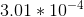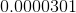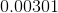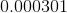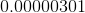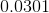Explanation:

Converting a value from scientific notation to standard notation is a simple matter of multiplication.Since we are always multiplying by a base ten term, the value of the exponent determines the increase/decrease in terms of orders of magnitude. In this case, an exponent ofindicates a four-fold decrease in order of magnitude. Some may find it easiest to determine the answer by simply adjusting the decimal four digits to the left, adding zeroes as necessary.

Whether you choose to multiply or to simply shift the decimal, the final answer will be.

### Example Question #1 : Scientific Notation And Significant Figures

Convert the given value to scientific notation, rounding to four significant figures.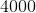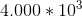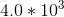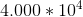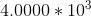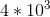Explanation:

Scientific notation is used to simplify exceptionally complex numbers and to quickly present the number of significant figures in a given value. The value is converted to an exponent form using base ten, such that only a single-digit term with any given number of decimal places is used to represent the significant figures of the given value. Non-significant zeroes can be omitted from the leading term, and represented only in the base ten exponent.

We are told that the final value must have four significant figures, so there will be four digits multiplied by the base ten term. Since the given value has only one significant figure, we will need to add three zeroes (4000).

To generate the single-digit leading term the decimal must be placed after the 4 (4.000). Then count the digits to the right of the decimal to determine the change in decimal placement (the decimal moves past three zeroes). Our ten will be raised to the power of three because there are three digits to the right of the final decimal placement.

Our final answer is.

### Example Question #31 : High School Chemistry

Convert the given value to scientific notation, rounding to four significant figures.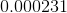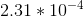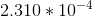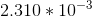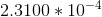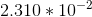Explanation:

Scientific notation is used to simplify exceptionally complex numbers and to quickly present the number of significant figures in a given value. The value is converted to an exponent form using base ten, such that only a single-digit term with any given number of decimal places is used to represent the significant figures of the given value. Non-significant zeroes can be omitted from the leading term, and represented only in the base ten exponent.

We are told that the final value must have four significant figures, so there will be four digits multiplied by the base ten term. Since the given value has only three significant figures, we will need to add a zero (2310).

To generate the single-digit leading term the decimal must be placed after the 2 (2.310). Then count the digits to the left of the decimal to determine the change in decimal placement (the decimal moves past three zeroes and the 2). Our ten will be raised to the power of negative four because there are four digits to the left of the final decimal placement.

Our final answer is.

### Example Question #1 : Scientific Notation And Significant Figures

Convert the given value to scientific notation, rounding to four significant figures.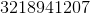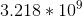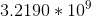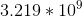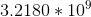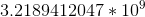Explanation:

Scientific notation is used to simplify exceptionally complex numbers and to quickly present the number of significant figures in a given value. The value is converted to an exponent form using base ten, such that only a single-digit term with any given number of decimal places is used to represent the significant figures of the given value. Non-significant zeroes can be omitted from the leading term, and represented only in the base ten exponent.

We are told that the final value must have four significant figures, so there will be four digits multiplied by the base ten term. We take only the first four digits, rounding the final digit depending on the final term; in this case we round up (3219).

To generate the single-digit leading term the decimal must be placed after the 3 (3.219). Then count the digits to the right of the decimal based on the original value to determine the change in decimal placement (the decimal moves past the following digits in the original term: 128941207). Our ten will be raised to the power of nine because there are nine digits to the right of the final decimal placement.

Our final answer is.

### Example Question #1 : Perform Operations With Numbers Expressed In Scientific Notation: Ccss.Math.Content.8.Ee.A.4

Convert the given value to scientific notation, rounding to six significant figures.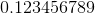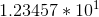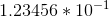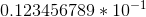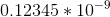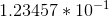Explanation:

Scientific notation is used to simplify exceptionally complex numbers and to quickly present the number of significant figures in a given value. The value is converted to an exponent form using base ten, such that only a single-digit term with any given number of decimal places is used to represent the significant figures of the given value. Non-significant zeroes can be omitted from the leading term, and represented only in the base ten exponent.

We are told that the final value must have six significant figures, so there will be six digits multiplied by the base ten term. We take only the first six digits, rounding the final digit depending on the final term; in this case we round up (123457).

To generate the single-digit leading term the decimal must be placed after the 1 (1.23457). Then count the digits to the left of the decimal to determine the change in decimal placement (the decimal moves past the 1 only). Our ten will be raised to the power of negative one because there is only one digit to the left of the final decimal placement.

Our final answer is.

← Previous 1

### All High School Chemistry Resources